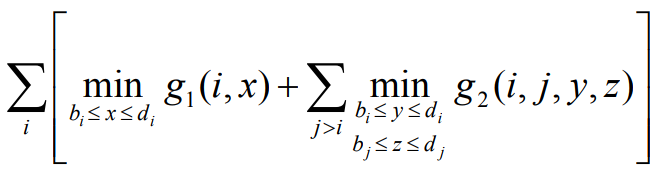Warning
This page is located in archive.

# Tutorial 11 - Protein structure, the MODELLER software

## Secondary structure prediction exercise

A simple, although not always reliable, way to discover the secondary structure of a peptide sequence is to look up a protein with similar primary sequence in a database. Let us try this! The task is to obtain the secondary structure of the following peptide sequence: HYLCKYVINAIPPTLTAKIHFRPELPAERNQLIQRLA

1. Go to https://blast.ncbi.nlm.nih.gov/Blast.cgi and click “Protein blast”.
2. Enter the sequence and enter “Homo sapiens (taxid:9606)” as organism.
3. Click the blast button and wait. This may take up to several minutes.
4. Look for the best matching protein. It should be: “monoamine oxidase A”
5. Enter this protein name to UniProt.
6. Check whether whether the result has a secondary sequence annotation and find the position respective to the BLAST match.

## Threading exercise

Recall the branch-and-bound threading algorithm from the lecture.

Suppose we have three segments (i, j, k), each of which includes three amino acids. For a given sequence there are three possible starting positions for each segment. (i ∈ {2,3,4}, j ∈ {8,9,10}, k ∈ {13,14,15}) We will be using the simple lower bound:Suppose that you are given the following values for the scores of the individual segments and the scores for segment interactions:

i j k
g1(i,2) = 5 g1(j,8) = 9 g1(k,13) = 3
g1(i,3) = 2 g1(j,9) = 7 g1(k,14) = 4
g1(i,4) = 8 g1(j,10) = 6 g1(k,15) = 1
i/j j/k i/k
g2(i,j,2,8) = 1g2(j,k,8,13) = 7g2(i,k,2,13) = 1
g2(i,j,2,9) = 2g2(j,k,8,14) = 8g2(i,k,2,14) = 2
g2(i,j,2,10) = 2g2(j,k,8,15) = 7g2(i,k,2,15) = 5
g2(i,j,3,8) = 5g2(j,k,9,13) = 1g2(i,k,3,13) = 5
g2(i,j,3,9) = 6g2(j,k,9,14) = 6g2(i,k,3,14) = 6
g2(i,j,3,10) = 4g2(j,k,9,15) = 8g2(i,k,3,15) = 4
g2(i,j,4,8) = 7g2(j,k,10,13) = 11g2(i,k,4,13) = 1
g2(i,j,4,9) = 3g2(j,k,10,14) = 12g2(i,k,4,14) = 2
g2(i,j,4,10) = 4g2(j,k,10,15) = 13g2(i,k,4,15) = 4

Using this information, compute the optimal threading.

## MODELLER overview

The purpose of this tutorial is to put our hands on a software for comparative protein structure modelling, namely the MODELLER. We will go through a basic tutorial for this software. At the end this tutorial, you should have a better understanding of what such software is capable of.

## Installation

Download the MODELLER version for your operating system from: https://salilab.org/modeller/download_installation.html (Note: It is also available from official repositories of some GNU/Linux distributions.)

You need to register yourself in order to obtain a license here: https://salilab.org/modeller/registration.html You should provide your university e-mail in the registration form.

## The turorial

We will follow this tutorial from the MODELLER webpages: https://salilab.org/modeller/tutorial/basic.html.

For those who are interested to learn more about MODELLER, there are also advanced tutorials: https://salilab.org/modeller/tutorial/

## See also

If you are interested, you may also have a look at the I-TASSER software.

A tutorial on homology modelling from the university of Frankfurt.

Branch and bound threading example taken from https://www.biostat.wisc.edu/bmi776/lectures/threading.pdf

courses/bin/tutorials/tutorial11.txt · Last modified: 2019/03/25 14:27 by barvijac